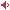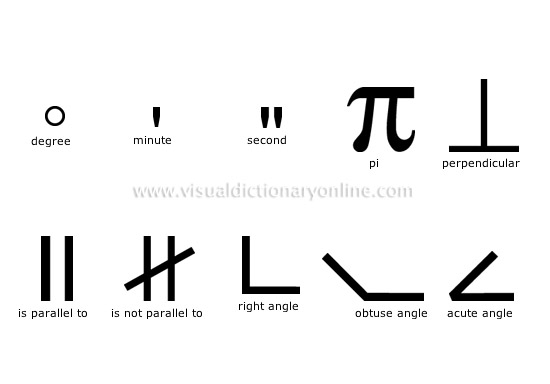Visual Dictionary Online

Theme

geometryMathematical discipline that studies the relations between points, straight lines, curves, surfaces and volumes.obtuse angleAngle between 90° and 180°.

acute angleAngle that is smaller than a right angle (less than 90°).

right angleAngle formed by two lines or two perpendicular planes that measures 90°.

perpendicularSymbol denoting that a straight line meets another at a right angle.

is not parallel toSymbol denoting that two straight lines do not remain at a constant distance from one other.

is parallel toSymbol denoting that two straight lines remain at a constant distance from one another.

minuteSymbol placed in superscript after a number that denotes degrees in sixtieths of a measure.

secondSymbol placed in superscript after a number that denotes degrees in sixtieths of a minute.

piConstant that represents the ratio of a circle’s circumference to its diameter; its value is approximately 3.1416.

degreeSymbol placed in superscript after a number to denote the opening of an angle or the length of an arc, or in front of an uppercase letter to identify a scale of measurement.